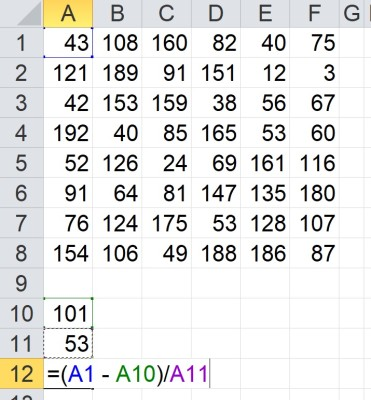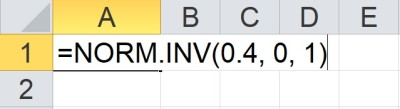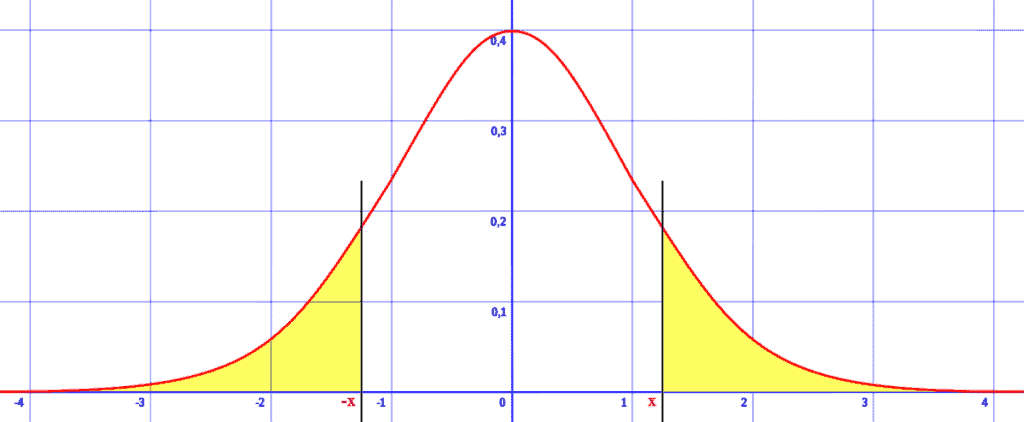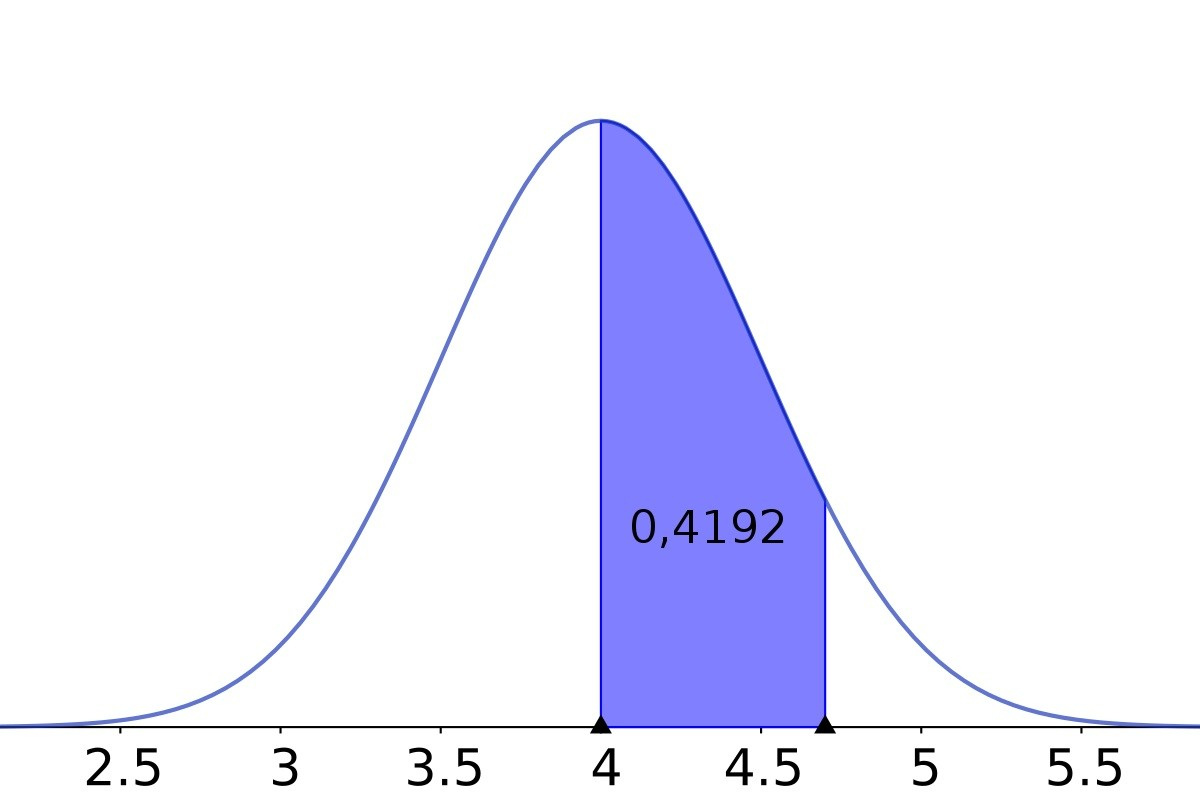# How To Find Z-Scores In Excel (Plus 5 Helpful Tips To Know)

Finding the z score for a value in a data set helps us to see how many standard deviations that number is from the mean by “standardizing” the distribution so the mean is 0 and the standard deviation is 1.  With a few steps, Microsoft Excel can find the z score of a given number for any set of values you choose as input.

So, how do you find z scores in Excel?  To find a z score in Excel, use the AVERAGE function to find the mean M. Next, use the STDEV.P function to find the standard deviation S. Then, find the z score of the value X with the formula Z = (X – M) / S. Both AVERAGE and STDEV.P take more arrays (cells, rows, columns, or blocks) as input.

Of course, z scores are also used with normal distributions for hypothesis testing and confidence intervals.

In this article, we’ll talk about how to find z scores in Excel.  We’ll also look at some examples to make the concept clear.

Let’s get started.

## How To Find Z-Scores In Excel

To find a z score (standard score) in Excel for a given value of X from a data set, we need to take a few steps:

You can see an example of how to do this below.

### Example: Find A Z-Score In Excel

Let’s say we have a data set of 48 values in the cells A1:F8, as shown below.

We want to find the z score for the value X = 43 (found in cell A1).

First, we calculate the mean in cell A10 with the formula

• =AVERAGE(A1:F8)

Next, we calculate the standard deviation in cell A11 with the formula

• =STDEV.P(A1:F8)

Then, we calculate the value of Z in cell A12 with the formula

• =(A1 – A10)/A11The formula =(A1 – A10)/A11 in cell A12 will calculate the z score of the value 43 (in cell A1), based on the mean and standard deviation of the data set.

This gives us a z score of -1.111.  So, X = 43 is 1.111 standard deviations below the mean.

The formula

• =NORM.DIST(A3, A10, A11, TRUE)

returns a value of 0.1293.  This means that the probability of getting a value of X <= 43 is 12.93%.

### Is There A Z Score Function In Excel?

There is no z score function in Excel.  If you like, you can use an add-on that provides this functionality, or you can write your own custom z score function in Excel.

The function could work in one of two ways:

• Take the x value, mean, and standard deviation as inputs, and return the z score as the output.
• Take the x value and a data set as inputs, and return the z score as the output.

In the second case, you would calculate the mean and standard deviation of the data set within the function.

In either case, you would use the mean and standard deviation to find the z score, according to the formula:

• Z = (X – M) / S

where M is the mean and S is the standard deviation of the data set.

However, note that Excel does have a Z.TEST function that can calculate the one-tailed p-value of a z-test.

So, in summary:

• To find the z value for a value of x in a given data set, use a custom Z score function.
• To find the z value for a probability in the standard normal distribution, use the NORM.INV function (see below for more details).

### How Do You Convert Probability To Z Score In Excel?

To convert a probability to a z score in Excel, use the NORM.INV function.  The inputs will be:

• The probability as the first input
• A mean of 0 as the second input
• A standard deviation of 1 as the third input

So, for a probability of P, the formula would look like this:

• =NORM.INV(P, 0, 1)

For example, a probability of 40% corresponds to z-score of -0.2533.

The following table shows some sample probabilities and the corresponding z scores.The Excel formula =NORM.INV(0.4, 0, 1) calculates the z score for a probability of 40% for a standard normal distribution (mean of 0, standard deviation of 1).

The following table shows just a few probabilities and the corresponding z scores for a normal distribution.

### How To Interpret Z Score

The z score Z = (X – M) / S from a given value of X in a data set tells you several things.

For example, the sign of z tells us whether the value of X is above or below average (mean):

• If z is positive (z > 0), then X is above average in the distribution (data set).
• If z is negative (z < 0), then X is below average in the distribution (data set).
• If z is zero (z = 0), then X is exactly average in the distribution (data set).  That is, X is equal to the mean.

The absolute value of z tells us how far the X value is from the mean.  In other words, a larger value of |z| means X is further away from the mean M (measured in terms of standard deviations S).

### When Is A Z Score Unusual?

A z score in a normal distribution is often considered unusual when z > 1.96 or z < -1.96.  For a normal distribution, these values are in the top 2.5% and bottom 2.5% of the distribution, respectively.Any value of z such that |z| > 1.96 is considered unusual. This means a value that is 1.96 or more standard deviations above or below the mean is unusual (5% chance total, or 2.5% chance on each side of the distribution).

Together, these values account for only 5% of the data in a normal distribution.  So, only 1 in 20 data values will have z values such that |z| > 1.96.

Of course, we can set any threshold we like for an “unusual” z score in a normal distribution, including:

• top or bottom 1%, that is, 1 in 100: (|z| > 2.326)
• top or bottom 0.1%, that is, 1 in 1,000: (|z| > 3.09)
• top or bottom 0.01%, that is, 1 in 10,000: (|z| > 3.719)
• top or bottom 0.001%, that is, 1 in 100,000: (|z| > 4.265)
• top or bottom 0.0001%, that is, 1 in 1,000,000: (|z| > 4.753)

### Is A Standardized Score Necessarily A Z Score?

The terms standard score, standardized score, and z score all mean the same thing.  That is, a z score does not assume a normal distribution.

When we standardize, the goal is to convert a distribution with a mean of M and a standard deviation of S to a distribution with a mean of 0 and a standard deviation of 1.This normal distribution has a mean of 4. We would need to subtract 4 from every value in the data set to get a distribution with a mean of 0.

To do this, we subtract the mean M from every data value and then divide by the standard deviation S.

So, the value X is standardized to the value Z = (X – M) / S.

However, it is important to remember that a z score is not necessarily a standard normal score.  A z score is only a standard normal score if the original distribution was a normal distribution.

Standardizing changes the mean and standard deviation of a distribution, but not its basic shape.  A distribution that is not normal will not be normal after standardizing.

### Does Z Score Change If Units Change?

The z score for a given value of X does not change if the units change.  Although X would change (along with M and S, the mean and standard deviation), the z score (X – M) / S would be unaffected.

Here is the proof:

Let’s say that we make a change of units for the variable X.  This would involve either addition, multiplication, or both.

For example:

• a conversion from feet to inches means we multiply by 12
• a conversion from Celsius to Fahrenheit means we multiply by 1.8 and add 32)A unit conversion, such as feet to inches, would change units but not the z score (the z score is unitless).

So, the new values in the distribution would look like aX + b, where a is not zero.

Multiplying by a would multiply the mean and standard deviation by a, so we would get aM and aS for the new mean and standard deviation.

Adding b would increase the mean by b and leave the standard deviation unchanged, so we would get aM + b and aS for the new mean and standard deviation

When we standardize, we would get a z score of:

• [(aX + b) – (aM + b)] / aS
• =[aX + b – aM – b] / aS
• =[aX – aM] / aS
• =a[X – M] / aS
• =[X – M] / S

This is the same z score we would get with the original distribution.  So, a change in units would not change the z score.

Remember that a z score is unitless, since the numerator (X and M) are in the same units as the denominator (S), so the units always cancel.

## Conclusion

Now you know how to find a scores in Excel, what they mean, and what they can tell you about a value in comparison to a data set.

You can learn about how to work with normal distributions in Excel here.

You can learn how to find mean in Excel here.

You can learn how to find median in Excel here.

You can learn how to find mode in Excel here.